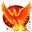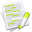#wx.TimeSpan¶

wx.TimeSpan class represents a time interval.

##Class Hierarchy¶Inheritance diagram for class TimeSpan:

##Methods Summary¶

 __init__ Default constructor, constructs a zero timespan. Abs Returns the absolute value of the timespan: does not modify the object. Add Adds the given wx.TimeSpan to this wx.TimeSpan and returns a reference to itself. Day Returns the timespan for one day. Days Returns the timespan for the given number of days. Format Returns the string containing the formatted representation of the time span. GetDays Returns the difference in number of days. GetHours Returns the difference in number of hours. GetMilliseconds Returns the difference in number of milliseconds. GetMinutes Returns the difference in number of minutes. GetSeconds Returns the difference in number of seconds. GetValue Returns the internal representation of timespan. GetWeeks Returns the difference in number of weeks. Hour Returns the timespan for one hour. Hours Returns the timespan for the given number of hours. IsEqualTo Returns True if two timespans are equal. IsLongerThan Compares two timespans: works with the absolute values, i.e. -2 hours is longer than 1 hour. IsNegative Returns True if the timespan is negative. IsNull Returns True if the timespan is empty. IsPositive Returns True if the timespan is positive. IsShorterThan Compares two timespans: works with the absolute values, i.e. 1 hour is shorter than -2 hours. Millisecond Returns the timespan for one millisecond. Milliseconds Returns the timespan for the given number of milliseconds. Minute Returns the timespan for one minute. Minutes Returns the timespan for the given number of minutes. Multiply Multiplies this time span by n. Neg Negate the value of the timespan. Negate Returns timespan with inverted sign. Second Returns the timespan for one second. Seconds Returns the timespan for the given number of seconds. Subtract Subtracts the given wx.TimeSpan to this wx.TimeSpan and returns a reference to itself. Week Returns the timespan for one week. Weeks Returns the timespan for the given number of weeks.

##Class API¶

class wx.TimeSpan(object)

Possible constructors:

TimeSpan()

TimeSpan(hours, min=0, sec=0, msec=0)


TimeSpan class represents a time interval.

### Methods¶

__init__(self, *args, **kw)Overloaded Implementations:

__init__ (self)

Default constructor, constructs a zero timespan.

__init__ (self, hours, min=0, sec=0, msec=0)

Constructs timespan from separate values for each component, with the date set to 0.

Hours are not restricted to 0-24 range, neither are minutes, seconds or milliseconds.

Parameters
• hours (long) –

• min (long) –

• sec (long) –

• msec (long) –

Abs(self)

Returns the absolute value of the timespan: does not modify the object.

Return type

wx.TimeSpan

Add(self, diff)

Adds the given wx.TimeSpan to this wx.TimeSpan and returns a reference to itself.

Parameters

diff (wx.TimeSpan) –

Return type

wx.TimeSpan

static Day()

Returns the timespan for one day.

Return type

wx.TimeSpan

static Days(days)

Returns the timespan for the given number of days.

Parameters

days (long) –

Return type

wx.TimeSpan

Format(self, format=DefaultTimeSpanFormat)

Returns the string containing the formatted representation of the time span.

The following format specifiers are allowed after %:

• H - Number of Hours

• M - Number of Minutes

• S - Number of Seconds

• l - Number of Milliseconds

• D - Number of Days

• E - Number of Weeks

• % - The percent character

Note that, for example, the number of hours in the description above is not well defined: it can be either the total number of hours (for example, for a time span of 50 hours this would be 50) or just the hour part of the time span, which would be 2 in this case as 50 hours is equal to 2 days and 2 hours.

wx.TimeSpan resolves this ambiguity in the following way: if there had been, indeed, the D format specified preceding the H , then it is interpreted as 2. Otherwise, it is 50.

The same applies to all other format specifiers: if they follow a specifier of larger unit, only the rest part is taken, otherwise the full value is used.

Parameters

format (string) –

Return type

string

GetDays(self)

Returns the difference in number of days.

Return type

int

GetHours(self)

Returns the difference in number of hours.

Return type

int

GetMilliseconds(self)

Returns the difference in number of milliseconds.

Return type

long

GetMinutes(self)

Returns the difference in number of minutes.

Return type

int

GetSeconds(self)

Returns the difference in number of seconds.

Return type

long

GetValue(self)

Returns the internal representation of timespan.

Return type

long

GetWeeks(self)

Returns the difference in number of weeks.

Return type

int

static Hour()

Returns the timespan for one hour.

Return type

wx.TimeSpan

static Hours(hours)

Returns the timespan for the given number of hours.

Parameters

hours (long) –

Return type

wx.TimeSpan

IsEqualTo(self, ts)

Returns True if two timespans are equal.

Parameters

ts (wx.TimeSpan) –

Return type

bool

IsLongerThan(self, ts)

Compares two timespans: works with the absolute values, i.e. -2 hours is longer than 1 hour.

Also, it will return False if the timespans are equal in absolute value.

Parameters

ts (wx.TimeSpan) –

Return type

bool

IsNegative(self)

Returns True if the timespan is negative.

Return type

bool

IsNull(self)

Returns True if the timespan is empty.

Return type

bool

IsPositive(self)

Returns True if the timespan is positive.

Return type

bool

IsShorterThan(self, ts)

Compares two timespans: works with the absolute values, i.e. 1 hour is shorter than -2 hours.

Also, it will return False if the timespans are equal in absolute value.

Parameters

ts (wx.TimeSpan) –

Return type

bool

static Millisecond()

Returns the timespan for one millisecond.

Return type

wx.TimeSpan

static Milliseconds(ms)

Returns the timespan for the given number of milliseconds.

Parameters

ms (long) –

Return type

wx.TimeSpan

static Minute()

Returns the timespan for one minute.

Return type

wx.TimeSpan

static Minutes(min)

Returns the timespan for the given number of minutes.

Parameters

min (long) –

Return type

wx.TimeSpan

Multiply(self, n)

Multiplies this time span by n.

Parameters

n (int) –

Return type

wx.TimeSpan

Returns

A reference to this wx.TimeSpan object modified in place.

Neg(self)

Negate the value of the timespan.

Return type

wx.TimeSpan

Negate(self)

Returns timespan with inverted sign.

Return type

wx.TimeSpan

static Second()

Returns the timespan for one second.

Return type

wx.TimeSpan

static Seconds(sec)

Returns the timespan for the given number of seconds.

Parameters

sec (long) –

Return type

wx.TimeSpan

Subtract(self, diff)

Subtracts the given wx.TimeSpan to this wx.TimeSpan and returns a reference to itself.

Parameters

diff (wx.TimeSpan) –

Return type

wx.TimeSpan

static Week()

Returns the timespan for one week.

Return type

wx.TimeSpan

static Weeks(weeks)

Returns the timespan for the given number of weeks.

Parameters

weeks (long) –

Return type

wx.TimeSpan